#### 期刊菜单

Bionic Design of Prosthetic Hand Based on Shape Memory Alloy
DOI: 10.12677/MOS.2023.122088, PDF , HTML, XML, 下载: 179  浏览: 274  国家自然科学基金支持

Abstract: Since most prosthetic hands are actuated by motors, which are large, heavy, and difficult to carry, this paper designs a modular SMA-actuators bionic prosthetic hand. Its quality is 188 g in total. Each finger can be actuated and controlled independently. By coiling with a pulley block, it increas-es the effective length of the SMA wire. A 3D model of the prosthetic hand is drawn, and kinematics analysis and simulation of the seven-link mechanism are carried out. Using MATLAB, this paper verifies the joint range of motion and kinematic trajectories of the fingertip. The control system is designed by taking STM32 as the controller and combing it with a multi-sensor. Through experi-ments, the motion state of the artificial finger is verified. According to the results, the output dis-placement of the SMA actuator can meet the driving distance requirements of the artificial finger, and the kinematic trajectories of the fingertip are consistent with the theoretical value which can achieve the expected goal of design. The research solves the problem of short actuated displace-ment of SMA and provides a design idea for using SMA as a new actuator and the mechanical struc-ture of bionic prosthetic hands.

1. 引言

2. 仿生假肢手设计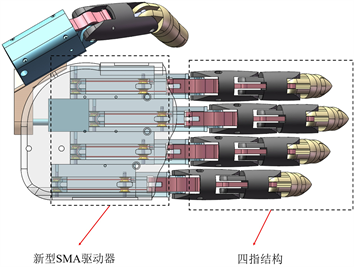Figure 1. Bionic hand 3D-model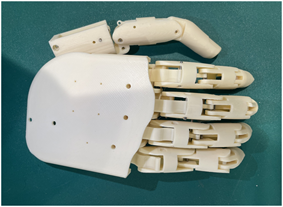Figure 2. Bionic hand physical prototype

2.1. 手指结构设计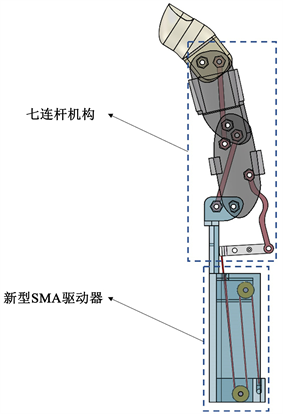Figure 3. Diagram of finger joint structure

2.2. SMA驱动器设计

2.2.1. SMA驱动器结构设计

2.2.2. SMA驱动器控制设计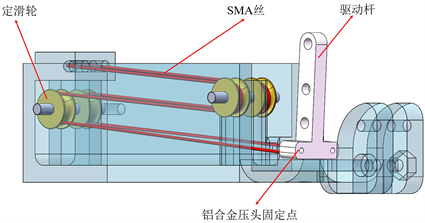Figure 4. Structure diagram of new SMA actuator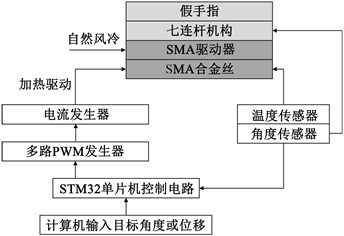Figure 5. Bionic prosthetic hand control system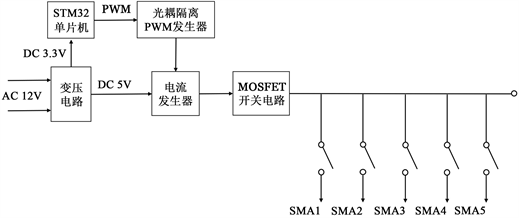Figure 6. Schematic circuit diagram

3. 假手指运动学分析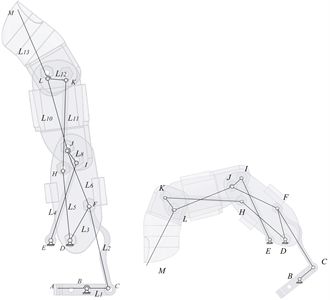Figure 7. Structure of single fingerTable 1. Design parameters for multi-link driving mechanism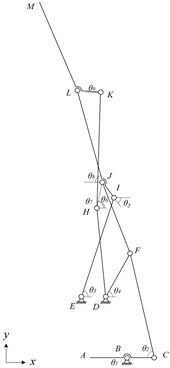Figure 8. Mechanism schematic diagram of finger

$\left\{\begin{array}{l}BC+CF=BD+DF\\ EI+IJ=ED+DJ\\ HJ+JL=HK+KL\end{array}$ (1)

$\left\{\begin{array}{l}{L}_{1}\mathrm{cos}{\theta }_{1}-{L}_{2}\mathrm{cos}{\theta }_{2}={L}_{3}\mathrm{cos}{\theta }_{4}-B{D}_{X}\\ {L}_{1}\mathrm{sin}{\theta }_{1}+{L}_{2}\mathrm{sin}{\theta }_{2}={L}_{3}\mathrm{sin}{\theta }_{4}+B{D}_{y}\\ {L}_{4}\mathrm{cos}{\theta }_{3}-{L}_{8}\mathrm{cos}{\theta }_{5}=E{D}_{x}-{L}_{DJ}\mathrm{cos}\left({180}^{\circ }-{\theta }_{4}-{\beta }_{1}\right)\\ {L}_{4}\mathrm{sin}{\theta }_{3}+{L}_{8}\mathrm{sin}{\theta }_{5}=E{D}_{y}+{L}_{DJ}\mathrm{sin}\left({180}^{\circ }-{\theta }_{4}-{\beta }_{1}\right)\\ {L}_{10}\mathrm{cos}\left(180+{\theta }_{5}-{\beta }_{4}\right)-{L}_{7}\mathrm{cos}\left({\beta }_{3}-180+{\theta }_{4}+{\beta }_{2}\right)={L}_{12}\mathrm{cos}{\theta }_{9}-{L}_{11}\mathrm{cos}{\theta }_{7}\\ {L}_{10}\mathrm{sin}\left(180+{\theta }_{5}-{\beta }_{4}\right)+{L}_{7}\mathrm{sin}\left({\beta }_{3}-180+{\theta }_{4}+{\beta }_{2}\right)={L}_{12}\mathrm{sin}{\theta }_{9}+{L}_{11}\mathrm{sin}{\theta }_{7}\end{array}$ (2)

$\left\{\begin{array}{l}{x}_{M}={L}_{9}\mathrm{cos}\left({\theta }_{5}+{\beta }_{5}\right)-{L}_{4}\mathrm{cos}{\theta }_{3}+{L}_{13}\mathrm{cos}\left({\beta }_{6}-{\theta }_{9}\right)-B{E}_{x}\\ {y}_{M}={L}_{9}\mathrm{sin}\left({\theta }_{5}+{\beta }_{5}\right)+{L}_{4}\mathrm{sin}{\theta }_{3}+{L}_{13}\mathrm{sin}\left({\beta }_{6}-{\theta }_{9}\right)+B{E}_{y}\end{array}$ (3)

$\left\{\begin{array}{l}{{x}^{\prime }}_{M}=-{L}_{9}\mathrm{sin}\left({\theta }_{5}+{\beta }_{5}\right){\omega }_{5}+{L}_{4}\mathrm{sin}{\theta }_{3}{\omega }_{3}+{L}_{13}\mathrm{sin}\left({\beta }_{6}-{\theta }_{9}\right){\omega }_{9}\\ {{y}^{\prime }}_{M}={L}_{9}\mathrm{cos}\left({\theta }_{5}+{\beta }_{5}\right){\omega }_{5}+{L}_{4}\mathrm{cos}{\theta }_{3}{\omega }_{3}-{L}_{13}\mathrm{cos}\left({\beta }_{6}-{\theta }_{9}\right){\omega }_{9}\end{array}$

$\left\{\begin{array}{l}{{{x}^{\prime }}^{\prime }}_{M}=-{L}_{9}\mathrm{cos}\left({\theta }_{5}+{\beta }_{5}\right){\omega }_{5}^{2}-{L}_{9}\mathrm{sin}\left({\theta }_{5}+{\beta }_{5}\right){\alpha }_{5}+{L}_{4}\mathrm{cos}{\theta }_{3}{\omega }_{3}^{2}\\ \text{}+{L}_{4}\mathrm{sin}{\theta }_{3}{\alpha }_{3}-{L}_{13}\mathrm{cos}\left({\beta }_{6}-{\theta }_{9}\right){\omega }_{9}^{2}+{L}_{13}\mathrm{sin}\left({\beta }_{6}-{\theta }_{9}\right){\alpha }_{9}\\ {{{y}^{\prime }}^{\prime }}_{M}=-{L}_{9}\mathrm{sin}\left({\theta }_{5}+{\beta }_{5}\right){\omega }_{5}^{2}+{L}_{9}\mathrm{cos}\left({\theta }_{5}+{\beta }_{5}\right){\alpha }_{5}-{L}_{4}\mathrm{sin}{\theta }_{3}{\omega }_{3}^{2}\\ \text{}+{L}_{4}\mathrm{cos}{\theta }_{3}{\alpha }_{3}-{L}_{13}\mathrm{sin}\left({\beta }_{6}-{\theta }_{9}\right){\omega }_{9}^{2}+{L}_{13}\mathrm{cos}\left({\beta }_{6}-{\theta }_{9}\right){\alpha }_{9}\end{array}$

SMA丝的位移Δl与驱动杆角度θ1之间的关系如下，其中R为驱动杆的旋转半径

$\mathrm{cos}{\theta }_{1}=\frac{2{R}^{2}-\Delta {l}^{2}}{2{R}^{2}}$ (4)

4. 实验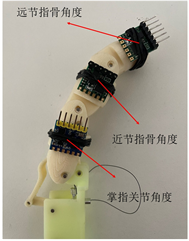(a) 各关节姿态传感器位置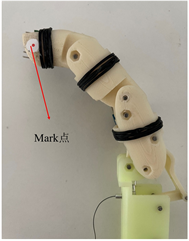(b) Mark点位置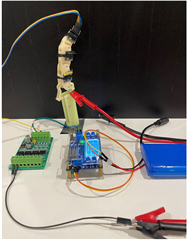(c) 实验平台搭建

Figure 9. Experiment platform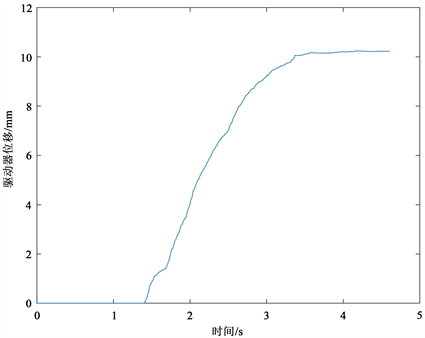Figure 10. Displacement changes of actuator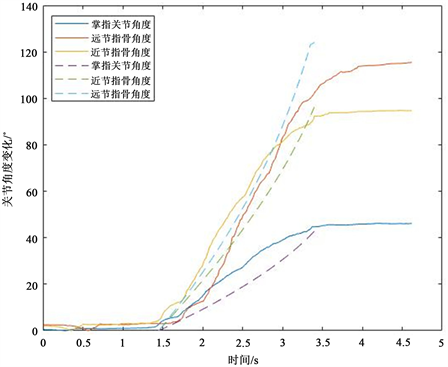Figure 11. Angle changes of finger joints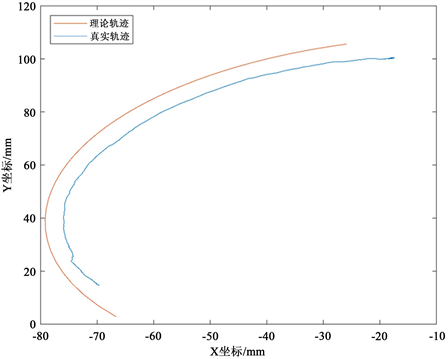Figure 12. Fingertip trajectory curve

5. 结论

NOTES

*通讯作者。

  中国残疾人联合会. 2010年末全国残疾人总数及各类[EB/OL]. 百度文库, 2012-06-26. http://wenku.baidu.com/view/97322d8802d276a200292e0f.html, 2013-03-22.  U, Z. and Todorov, E. (2016) Design of a Highly Biomimetic Anthropomorphic Robotic Hand towards Artificial Limb Regeneration. Proceedings of the 2016 IEEE International Conference on Robotics and Automation (ICRA), Stockholm, 16-21 May 2016, 3485-3492.  Kurita, Y., Ono, Y., Ikeda, A. and Ogasawara, T. (2011) Human-Sized Anthropomorphic Robot Hand with Detachable Mechanism at the Wrist. Mechanism and Machine Theory, 46, 53-66. https://doi.org/10.1016/j.mechmachtheory.2010.08.011  Grebenstein, M., Albu-schaffer, A., Bahls, T., et al. (2011) The DLR Hand Arm System. IEEE International Conference on Robotics and Automation, Shanghai, 9-13 May 2011, 3175-3182. https://doi.org/10.1109/ICRA.2011.5980371  Rothling, F., Haschke, R., Steil, J.J. and Ritter, H. (2007) Platform Portable Anthropomorphic Grasping with the Bielefeld 20-DOF Shadow and 9-DOF TUM Hand. Proceedings of the 2007 IEEE/RSJ International Conference on Intelligent Robots and Systems, San Diego, 29 October-2 November 2007, 2951-2956.  Kargov, A., Werner, T., Pylatiuk, C. and Schulz, S. (2008) Development of a Miniaturised Hydraulic Actua-tion System for Artificial Hands. Sensors and Actuators A: Physical, 141, 548-557. https://doi.org/10.1016/j.sna.2007.10.025  Park, C., et al. (2019) Shape Memory Alloy-Based Spring Bundle Actuator Controlled by Water Temperature. IEEE/ ASME Transactions on Mechatronics, 24, 1798-1807. https://doi.org/10.1109/TMECH.2019.2928881  Ning, G., et al. (2021) A Bionic Soft Tongue Driven by Shape Memory Alloy and Pneumatics. Bioinspiration & Biomimetics, 16, Article ID: 055008.  Lohse, F., Wende, C., et al. (2021) Bio-Inspired Semi-Flexible Joint Based on Fibre-Reinforced Composites with Shape Memory Alloys. Journal of Intelligent Material Systems and Structures, 32, 462-472. https://doi.org/10.1177/1045389X20959460  Ye, T., Wang, Y., Xu, S., Wang, Y. and Li, J. (2022) Modeling and Motion Control of an Octopus-Like Flexible Manipulator Actuated by Shape Memory Alloy Wires. Journal of Intelligent Mate-rial Systems and Structures, 33, 3-16. https://doi.org/10.1177/1045389X211023579  吕雨微, 芦吉云, 王宜耀. 双程SMA丝驱动的柔性弯曲关节设计与测试[J]. 机械科学与技术, 2022, 41(9): 1334-1339.  Zhang, J., Cong, M., Liu, D., et al. (2022) A Lightweight Varia-ble Stiffness Knee Exoskeleton Driven by Shape Memory Alloy. Industrial Robot, 49, 994-1007. https://doi.org/10.1108/IR-11-2021-0262  Jian, L., He, J.M., et al. (2019) A Biomimetic Flexible Fishtail Embedded with Shape Memory Alloy Wires. IEEE Access, 7, 166906-166916.  Terrile, S., Miguelaez, J. and Barrientos, A. (2021) A Soft Haptic Glove Actuated with Shape Memory Alloy and Flexible Stretch Sensors. Sensors, 21, 5278. https://doi.org/10.3390/s21165278  Bacalangco, J., Bawagan, K., et al. (2021) Design and Control of Robotic Elbow Using Shape Memory Alloy as Actuators. 2021 6th International Conference on Communication and Electronics Systems (ICCES), Coimbatre, 8-10 July 2021, 13-20. https://doi.org/10.1109/ICCES51350.2021.9489192  Muralidharan, M. and Palani, I. (2021) Development of Subcarangiform Bionic Robotic Fish Propelled by Shape Memory Alloy Actuators. De-fence Science Journal, 71, 94-101. https://doi.org/10.14429/dsj.71.15777  Andrianesis, K. and Tzes, A. (2015) De-velopment and Control of a Multifunctional Prosthetic Hand with Shape Memory Alloy Actuators. Journal of Intelligent & Ro-botic Systems, 78, 257-289. https://doi.org/10.1007/s10846-014-0061-6  孙茂凱, 陈羿宗, 王生海, 韩广冬, 徐小清, 陈海泉. 形状记忆合金驱动的软体仿生手掌[J]. 科学技术与工程, 2021, 21(34): 14618-14624.  Deng, E., et al. (2021) A Soft 3D-Printed Ro-botic Hand Actuated by Coiled SMA. Actuators, 10, 6. https://doi.org/10.3390/act10010006  郝丽娜, 郭少飞, 陈洋. SMA驱动的模块化仿人手指设计与研究[J]. 东北大学学报(自然科学版), 2017, 38(8): 1137-1141.  Erol, A., Bebis, G., Nicolescu, M., et al. (2005) A Review on Vi-sion-Based Full DOF Hand Motion Estimation. IEEE Computer Society Conference on Computer Vision and Pattern Recogni-tion, San Diego, 21-23 September 2005, 75. https://doi.org/10.1109/CVPR.2005.395  Kim, H.I., Han, M.W., Song, S.H., et al. (2016) Soft Morphing Hand Driven by SMA Tendon Wire. Composites Part B: Engineering, 105, 138-148. https://doi.org/10.1016/j.compositesb.2016.09.004